Algebra 1 : Systems of Inequalities

Example Questions

Example Question #71 : Systems Of Inequalities

Solve the inequality: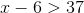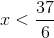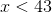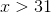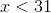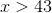Explanation:

In order to solve the inequality, we will need to add six on both sides.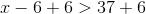Simplify both sides of the equation.

The answer is:Example Question #72 : Systems Of Inequalities

Solve the inequality: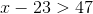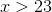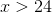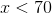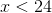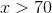Explanation:

In order to solve for x, add 23 on both sides of the equation.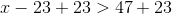Simplify both sides.

The answer is:Example Question #73 : Systems Of Inequalities

Solve the inequality: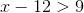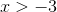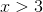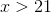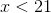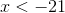Explanation:

In order to solve this inequality, add 12 on both sides.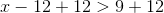Simplify both sides of the equation.The answer is:Example Question #74 : Systems Of Inequalities

Solve the inequality: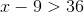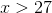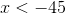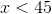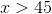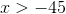Explanation:

To isolate the x variable, add nine on both sides of the equation.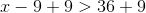Simplify both sides of the equation.The answer is:Example Question #75 : Systems Of Inequalities

Solve the inequality: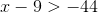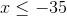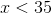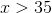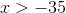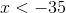Explanation:

In order to solve this inequality, add nine on both sides.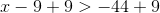Simplify both sides of the inequality.The answer is:Example Question #76 : Systems Of Inequalities

Solve the inequality: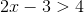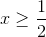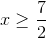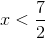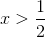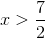Explanation: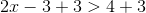Simplify both sides.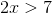Divide by two on both sides.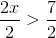Simplify both sides.

The answer is:Example Question #77 : Systems Of Inequalities

Solve the inequality: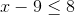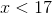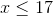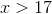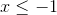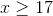Explanation:

To solve this inequality, simply add nine on both sides.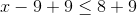Simplify both sides of the inequality.The answer is:Example Question #78 : Systems Of Inequalities

Solve the inequality: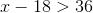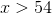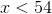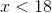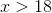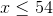Explanation:

In order to solve this inequality, add 18 on both sides.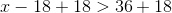Simplify both sides.The answer is:Example Question #79 : Systems Of Inequalities

Solve the inequality: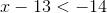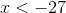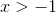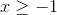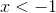Explanation:

Addon both sides.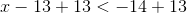Simplify both sides. The sign does not change.

The answer is:Example Question #80 : Systems Of Inequalities

Solve the inequality: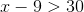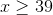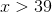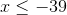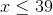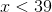Explanation:

In order to find the solution to this inequality, add nine on both sides.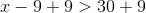Simplify both sides.  There is no need to change the direction of the sign.

The answer is: